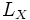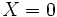# Ricci soliton

This article defines a property that makes sense for a Riemannian metric over a differential manifold

This property of a Riemannian metric is Ricci flow-preserved, that is, it is preserved under the forward Ricci flow

## Definition

### Definition in terms of a vector field

A Riemannian metric$g$ on a differential manifold$M$ is termed a Ricci soliton if it satisfies the following:$L_X(g) + 2\lambda g = -2R(g)$

at all points$x$ and all times$t$.

Here:

•$X$ is a suitable complete vector field on$M$ and$L_X$ denotes the Lie derivative with respect to$X$
•$\lambda$ is a suitable real number
•$R(g)$ denotes the Ricci curvature tensor of$g$.

In general, there may be more than one$X$ giving the same Ricci soliton.

### Definition in terms of the Ricci flow

A Ricci soliton is a point in the space of Riemannian metrics whose trajectory is a self-similar solution to the Ricci flow. In other words, if we look at the Ricci flow on the moduli space obtained on quotienting both by diffeomorphism equivalence and by conformal equivalence, its image is a fixed point in the Ricci flow on that.

## Relation with other properties

### Stronger properties

• Ricci-flat metric: Take$\lambda = 0, X = 0$
• Einstein metric: Take$X = 0$ and$\lambda$ as the negative of the cosmological constant of the Einstein metric.

### Properties of solitons

Ricci solitons are such an important class of metrics that we also talk of properties of solitons (which actually just means properties of metrics satisfied by solitons), such as: